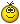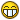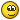# Thread: Cyrillic letters and their Roman opposite

1. ## Cyrillic letters and their Roman opposite

Hi, i'm just into 'self-learning' russian, because this is my last year in school and I won't have the time to take russian-lessons by now. so i try to get some pre-knowledge and right know i'm looking for the roman opponents of the cyrillic letters. could you correct me if there are mistakes?

A = A

б = i

B= B

г = G

д = D

E= E

Ж = Z (?)

З = Z (?)

и = Y ('J' in non english?)

K = K

л = L

M = M

н = N

O = O

П = P

P = R

C = S

т = T

Y = ???

ф = F

X = ???

ц = ???

ч = ???

ш = ???

щ = ???

ь = V

ы = ???

э = E

ю = Yu(?)

я = Ya(?)

Thanks for your help and i'd appreciate if you take some time and correct it or tell me where i can find a list. Maybe someone is so kind and leaves his Email or MSN-address, because I could need some tips to start efficently learning.

regarts,
tim

(my msn and mail is dirrtystyle@gmx.de ! )Reply With Quote

2. A = A

б = B

B= V

г = G

д = D

E= ye

Ж = zh (or like the 's' in 'pleasure')

З = Z

и = EE

K = K

л = L

M = M

н = N

O = O

П = P

P = R

C = S

т = T

Y = U (oo)

ф = F

X = CH in Scot's 'loch', or German 'Bach'

ц = TS

ч = CH

ш = SH

щ = long SH, but there will be an argument about this oneь = Soft sign, doesn't have a sound of its own, it modifies the preceding consonant by making it soft.

ы = I (English doesn't have this sound, but it's closest to the 'i' in 'ill'. You'll need to hear it pronounced by a native to get it though, text explainations don't work (see also: щ )

э = E

ю = Yu (As in 'Universe')

я = Ya

Oh, and you missed a couple:

ё = Yo

ъ = Hard sign. Same deal as with the soft sign, but makes preceding consonant hard.

й = short и. Acts as a 'glide' when attached to another vowel, in the same way the 'y' in English 'boy'Reply With Quote

3.good job.

At the beginning is very important to know it.Reply With Quote

####Posting Permissions

• You may not post new threads
• You may not post replies
• You may not post attachments
• You may not edit your posts
•